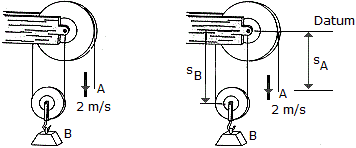# Engineering Mechanics - Kinematics of Particle (KOP) - Discussion

### Discussion :: Kinematics of Particle (KOP) - General Questions (Q.No.12)

12.If the end of the cable at A is pulled down with a speed of 2 m/s, determine the speed at which block B arises.

 [A]. vB = 4.00 m/s[B]. vB = 1.000 m/s[C]. vB = 1.000 m/s[D]. vB = 4.00 m/sExplanation:

No answer description available for this question.

 Eno said: (Sep 7, 2019) According to the number of strings in pulley B and the side A. The equation of dependency would be: XA+2XB= C. : . VA + 2VB =0, : . VB=-VA /2, VB = -2/2 =-1m/s. But the direction if the positive velocity VA is downwards, therefore, the negative velocity would be upward, So, the answer is B.